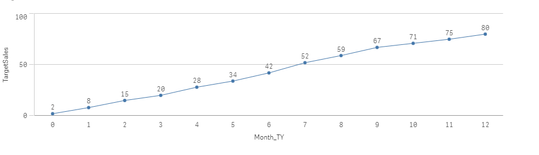# New to Qlik Sense

Discussion board where members can get started with Qlik Sense.

Announcements
Coming your way, the Qlik Data Revolution Virtual Summit. October 27-29. REGISTER
cancel
Showing results for
Did you mean:
HighlightedContributor III

## Target Line

Hi,

I need to create a 'Target' line within my line chart based on the 'Month_TY' , but not quite sure how to do it.

The X axis ('Month_TY') shows 0 - 12. What  I would like to see on my line chart is the following values if possible please? Example: month 0, the target of new sales is 2, so if my actual sales are 5, I can clearly see we have beaten the target put in place.

if 'Month_TY' = 0 then 2

if 'Month_TY' = 1 then 8

if 'Month_TY' = 2 then 15

if 'Month_TY' = 3 then 20

if 'Month_TY' = 4 then 28

if 'Month_TY' = 5 then 34

if 'Month_TY' = 6 then 42

if 'Month_TY' = 7 then 52

if 'Month_TY' = 8 then 59

if 'Month_TY' = 9 then 67

if 'Month_TY' = 10 then 71

if 'Month_TY' = 11 then 75

if 'Month_TY' = 12 then 80

Any idea if this is possible please?

2 Solutions

Accepted Solutions
HighlightedMaster III

Maybe you can try with the nested if conditions

if(Month_TY = 0,2,
if(Month_TY = 1, 8,
if(Month_TY = 2,15,
if(Month_TY = 3,20,
if(Month_TY = 4,28,
if(Month_TY = 5,34,
if(Month_TY = 6,42,
if(Month_TY = 7,52,
if(Month_TY = 8,59,
if(Month_TY = 9,67,
if(Month_TY = 10,71,
if(Month_TY = 11,75,
if(Month_TY = 12,80))))))))))))) as field

we can do the same using Applymap

HighlightedCreator II

hi @thomas_2583 ,

you can use nested if and you can also create a inline table  below:

Target:
Month_TY, TargetSales
0,2
1,8
2,15
3,20
4,28
5,34
6,42
7,52
8,59
9,67
10,71
11,75
12,80
];

output:regards,

raji

2 Replies
HighlightedMaster III

Maybe you can try with the nested if conditions

if(Month_TY = 0,2,
if(Month_TY = 1, 8,
if(Month_TY = 2,15,
if(Month_TY = 3,20,
if(Month_TY = 4,28,
if(Month_TY = 5,34,
if(Month_TY = 6,42,
if(Month_TY = 7,52,
if(Month_TY = 8,59,
if(Month_TY = 9,67,
if(Month_TY = 10,71,
if(Month_TY = 11,75,
if(Month_TY = 12,80))))))))))))) as field

we can do the same using Applymap

HighlightedCreator II

hi @thomas_2583 ,

you can use nested if and you can also create a inline table  below:

Target:
Month_TY, TargetSales
0,2
1,8
2,15
3,20
4,28
5,34
6,42
7,52
8,59
9,67
10,71
11,75
12,80
];

output:regards,

raji